# The Trinity of Inaccuracy: Phase Noise, Jitter and Short-Term Stability – What Everyone Should Know About Their Measurement and Interrelationships

January 10, 2023

In electrical components and circuits, noise effects with different physical causes occur everywhere. In crystal oscillators there are three primary noise generating mechanisms: A ubiquitous background noise due to the thermal motion of the atoms and molecules of all components creates an insurmountable noise floor, which mainly affects noise far from the carrier (white noise). Noise caused by semiconductor components is called shot noise which has a 1/f dependence on the frequency. The dominant noise source close to the carrier is called flicker noise, which largely depends on the quality of the crystal. A high Q-factor of the crystal suppresses noise near the carrier. Depending on the application, noise is described differently; phase noise, jitter and short-term stability are different ways of looking at the same physical phenomena. This article provides an overview of the interrelationships.

Ideal oscillators produce a time-dependent, sinusoidal output voltage of the form:with amplitude A0 and frequency f0. This sine wave has a perfect period, and the Fourier transform of u(t) is a spectrally pure delta function δ(f-f0). A non-ideal, noisy signal u’(t) can be described in general terms by introducing a term for the amplitude noise (t) and a term for the phase noise Δφ (t) of the signal in the time domain given by:Here A0 is the amplitude of the pure sinusoidal signal and f0 its nominal fundamental frequency, which can be interpreted as a statistical mean value. In this case, the frequency spectrum is no longer spectrally pure, but a function of frequency, or more precisely, the Fourier transform of the time-dependent voltage signal.Figure 1 Pure sinusoidal waveform (a) and effect of a random, time-dependent phase deviation = δφ.

Figure 1 shows the short-term frequency instabilities caused by the phase noise term. They show up in the time domain as a deviation of the zero crossings (phase angle) of the actual signal waveform compared to the ideal sinusoid. A modulation of the amplitude is not shown in this figure.

In the following discussion, only the temporal change of the phase angle is considered, which is caused by random, and thus Gaussian distributed processes. In the following, the three most important forms of description, namely phase noise L(f), jitter ΔT(Δf) and short-time stabilityare described.

PHASE NOISE

Considering the phase instability of a signal source as phase noise is one of the possible representations to be considered in this article. The amplitude of the phase noise signal L(f), more precisely the noise power spectral density of the phase noise, increases with increasing proximity to the carrier. An empirical description of the single-sideband phase noise is given by the so-called Leeson formula. It describes the amplitude of the phase noise as a function of carrier frequency f0 of the oscillator, its quality factor Ql, cutoff frequency fc, noise factor of the amplifier F, Boltzmann constant kB, absolute temperature T and output power Ps: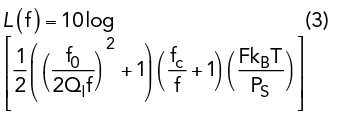Without having to analyze this equation in detail, it is easy to estimate the behavior of phase noise for small and large frequencies f. For small frequency distances from the carrier, the two factorsandgrow. Due to the monotonic property of the logarithm, L(f) also grows. Far away from the carrier, for f ∞, these two terms vanish and the phase noise tends toward the value.1

Therefore, it is very important that a requirement for maximum phase noise includes the exact distance from the carrier at which a specific value of the phase noise is specified. The fraction close to the carrier is largely determined by the Q of the crystal, where the phase noise fraction for frequencies above 1 kHz is determined by the noise of the semiconductor devices used.

In principle, the phase noise of an oscillator can always be measured (approximately) directly by means of a spectrum analyzer if the local oscillator of the measuring device has a significantly better phase noise performance than the device under test (DUT) and the sampling rate is far above the Nyquist frequency of the signal. Spectral analysis using a fast Fourier transform (FFT) can be used to determine the spectral distribution of the noise far away from the carrier frequency. Here, however, the measurement is limited by the noise characteristics and the limited sampling rate of the spectrum analyzer.

In the following, a much more precise measurement method is presented, which also enables the measurement of DUTs with extremely low phase noise. This method is generally valid and suitable for oscillators with high phase noise as well. To determine phase noise, the so-called phase detector method with phase locked loop (PLL) synchronization of the reference phase is used.Figure 2 PLL measurement for determining the phase noise of an oscillator (DUT).

As shown in Figure 2, this method uses a quadrature mixer as a phase detector. Here, the reference oscillator and the mixer form a PLL. By manipulating the control voltage of the reference oscillator, a condition is sought in which the signal of the test oscillator (DUT) and the signal of the reference oscillator have a phase shift ofwith respect to each other at the same frequency. Both signals are mixed in a mixer element. In general, for given input signals fa and fb, the output signal of a mixer can be described as a superposition of the high frequency sum signal (fa+fb) and the low frequency difference signal |fa- fb|.

The unwanted sum signal at the output of the mixer is removed by means of a lowpass filter, so that only the difference signal remains. The low frequency signal component filtered in this way generates a time-dependent voltage v(t) whose maximum amplitude is determined by the scaling factor of the phase detector constant KD. In general, KD is device-dependent and has no influence on the calculation of the phase noise L(f).Considering the small-angle approximation cos() for <<1, Equation (4) can be simplified, assuming a mean phase difference of.The output voltage v(t) of the phase comparator is evaluated as a measure of the phase changes in the time domain. The calculation of the single sideband phase noise L(f) is performed by means of an FFT in the frequency domain. If the reference oscillator has the same phase noise characteristics as the test oscillator, the measurement result must be corrected by -3 dB (factor of 0.5), since the uncorrelated noise powers of both oscillators add up. If the reference oscillator has a better phase noise by at least one decade, no correction is necessary.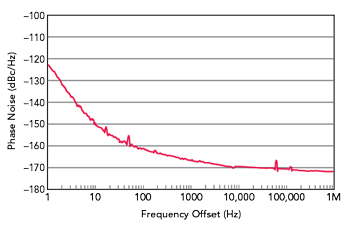Figure 3 Phase noise measurement of an ultra-low phase noise oscillator with a carrier frequency of 10 MHz.

Figure 3 is an example of a phase noise measurement of an ultra-low phase noise oscillator from KVG Quartz Crystal Technology with a carrier frequency of 10 MHz. The measurement was performed with a Holzworth phase noise analyzer with a measurement time of 1 hour. Both the near-carrier phase noise with a value of -122.5 dBc/Hz at 1 Hz offset and the phase noise of -169 dBc/Hz at 10 kHz show the limits of what is physically feasible.

A standard oven-controlled crystal oscillator (OXCO) at the same frequency has about 20 dBc/Hz worse phase noise at 1 Hz carrier offset than an ultra-low phase noise oscillator. Phase noise measurements are strongly sensitive with respect to mechanical or electromagnetic disturbances originating from the environment of the measurement setup. In Figure 3, small disturbances of the phase noise measurement can be observed, for example, at 50 Hz (mains frequency household current) and 16.7 Hz (mains frequency of the German railway), which can be further minimized by suitable mains filters of the measurement equipment and the control voltage of the oscillator.

JITTER

In addition to phase noise, jitter is another representation of the deviation of the phase angle of a periodic signal. It can be graphically interpreted as the time deviation of a signal from its ideal position over a series of signal periods. With an ideal measuring device (infinite time resolution and no inherent noise), one could look at individual clock edges and derive a jitter value via an absolute time measurement. This is not possible, however, even with the best measuring devices available.

In practice, the jitter measurement is often derived from the phase noise measurement, previously described, and will be explained in more detail later. For a direct jitter measurement, a measurement by means of a so-called “eye diagram” is used, in which the measurement is performed relative to a reference edge.Figure 4 Jitter measurement using a delayed signal.

The eye diagram consists of a superposition of many sections from the signal curve to be measured. An exemplary measurement setup is shown in Figure 4. The signal from the DUT is split by a splitter with one signal path fed through a delay line whose delay is greater than the period of the signal. The non-delayed signal serves as a trigger and the delayed signal is applied to the input of the oscilloscope. This results in the phase shift always being measured relative to one of the preceding signal edges. By superimposing a large number of these measurements, a quantitative value of the jitter magnitude can be determined from the “opening of the eye diagram” by means of statistical analysis.Figure 5 Deriving jitter from the eye diagram.

Figure 5 is an example of an eye diagram. Due to the intrinsic signal trigger, the signal edges are mapped on top of each other according to their phase shifts. From the superposition of several oscillation periods, quantities such as the average period duration and jitter can be determined. From the width of the signal distribution at zero crossing, a quantitative value for the jitter can be calculated on a statistical basis. In this example, the peak-to-peak value of the jitter is shown as the maximum expansion of the signal curve.

This measurement method is limited by the noise of the oscilloscope below the frequency range f = 1/(2 π τd), where τd is the length of the delay line. Below this cutoff frequency, the sensitivity drops by about 20 dB per decade. Thus, this method is well suited for jitter measurements at frequencies far from the carrier, for example the sidebands.

The noise in oscillators is basically a superposition of stochastic processes. The peak value is therefore usually specified on a purely statistical basis using a Gaussian distribution as the distribution function. This results in a crest factor (ratio of peak value to RMS value) of 3 between the peak and RMS value, or factor of 6 between the peak-to-peak and RMS value. The maximum jitter amplitude is with a probability of 99.7 percent (deviation in the interval ±3σ) within the statistical limits of the specified peak-to-peak value. When measuring and specifying jitter, it is important to note whether the specification is expressed as the effective value (RMS), peak value (peak) or peak-to-peak value (peak-to-peak).

SHORT-TERM STABILITY

Along with phase noise and jitter, short-term stability can be used to describe phase deviations of a signal. The Allan deviation, named after the physicist D.W. Allan, is used as a common measure of short-term stability. The Allan deviation (ADEV) is defined as the square root of the Allan variance σ2 (τ):Here, the Allan variance itself is defined as half of the expected value of the difference squares of two consecutive measured values (denoted here as yn and yn+1) of the normalized frequency deviation in the time interval τ. It should be noted that the ADEV is a function of the averaging time τ, which defines the period in which the average of the expected value is calculated.Compared to the classical variance, where the deviation from the mean is measured in each case, the Allan variance only considers the deviation of two successive measured values. This leads to a convergence for all kinds of noise even with long averaging times with a mean value drift (e.g., random walk processes).

The measurement of short-term stability in the form of the ADEV can be carried out in different ways. For low frequency signals and low short-term stability, the frequency deviation between two successive oscillation periods can be determined directly by an absolute time measurement. In the following, one of many possible measurement methods is presented, which is also suitable for the measurement of high precision signals.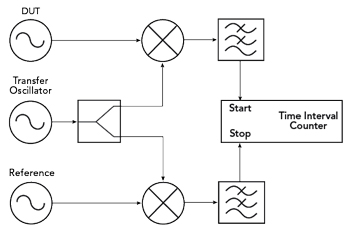Figure 6 Determining the short-term stability using a dual mixed signal.Figure 7 ADEV measurement of a 10 MHz ultra-low phase noise oscillator.

As shown in Figure 6, the dual mixer time difference method requires a reference oscillator and a so-called transfer oscillator in addition to the DUT. In this simple example, the reference oscillator must have better short-term stability than the DUT. During the measurement the transfer oscillator is slightly detuned against the other two signal sources. Here, a detuning of a few Hz up to a few kHz gives the best results, depending on the application.

By mixing this signal with the two signals of the DUT and the reference and subsequent lowpass filtering, two low frequency signals are produced. These signals are used as start and stop triggers for a time interval counter. With the interval counter the time difference between the zero crossings of both signals is measured. Depending on the desired averaging time τ, an adequate frequency offset of the transfer oscillator must be set.

If the short-term stability of the reference oscillator is not significantly better than that of the DUT, this can no longer be neglected. This effect can be mathematically corrected by using another reference oscillator. For this purpose, we refer to the so-called “three-cornered hat method.”2

Figure 7 is an example of an ADEV measurement of an ultra-low phase noise oscillator from KVG Quartz Crystal Technology with a carrier frequency of 10 MHz. Because the DUT has very good short-term stability, the measurement was performed using the three-cornered-hat method. Two oscillators with 10 MHz each were used as references. The total measurement duration was 4 hours, the sample interval was 0.1 s. The test oscillator achieved an ADEV of 1.8 × 10-13 at a time interval of τ=1 s.

RELATIONSHIP OF THE DIFFERENT REPRESENTATION TYPES

Phase Noise and Jitter

As previously described, phase noise and jitter are two different ways of describing the same physical signal property; therefore, it is obvious to look for possibilities to relate them. Jitter within a defined frequency range can be calculated from the measured values of phase noise by integrating L(f) over the frequency. The jitter power P in the frequency interval f1 to f2 is defined for a given single-sideband phase noise L(f) as:It should be noted that the phase noise is normalized by a factor of 2πf0, where f0 represents the carrier frequency. This also ensures the correct conversion of units.

The RMS value of jitter ΔT(Δf) in the specified frequency range can be calculated as the square root of the jitter power P: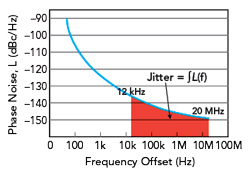Figure 8 Jitter can be calculated by integrating the phase noise over a frequency interval.3,4

As previously mentioned, this conversion provides another indirect way of measuring jitter. Often in practice, phase noise is determined for a typical offset range from 1 Hz to about 10 MHz. From this, the resulting jitter value can then be flexibly calculated by integration over different frequency ranges (see Figure 8).

A reverse calculation of phase noise from measured values of jitter over certain bandwidths is mathematically not possible, since for this the jitter would have to be known for all possible bandwidths/frequency intervals.

Phase Noise and Short-Term Stability

Short-term stability can also be determined mathematically from the values of L(f). The transition from phase noise, which is a function of frequency, to ADEV, which is a function of time, can be described by means of a Fourier transform. The derivation of this transform is far beyond the scope of this article, so reference is made to Barnes et al.5

As a result, the Allan variance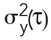can be written as an integral over the entire frequency range with carrier frequency f0, averaging time τ and frequency offset f as integration variables.The property from Equation (10) imposes certain conditions on the spectral distribution of the phase noise, for example, that the magnitude of the phase noise becomes very small for large distances from the carrier. These constraints also go very deep mathematically, so no further consideration is given.

SUMMARY

The need for high precision frequency sources, such as quartz oscillators, with extremely low noise characteristics is undisputed for modern technology of the 21st century. Whether it be measurement technology, data transmission or navigation—in all areas there are applications that place the highest demands on signal sources.

Depending on the field of application, a different one of the described measurement quantities is proven to be practical. In telecommunications, the specification of a maximum jitter value is usually resorted to, since it can be used to derive how high the bit error rate is during the transmission of discrete information. In metrology, on the other hand, the oscillator is often characterized by a phase noise curve, in which the phase noise values are specified at different distances from the carrier signal depending on whether phase noise close to or far from the carrier is relevant for the specific application.

Since all approaches are based on the same physical phenomenon, a conversion from one form of representation to the other can be performed if the data basis is sufficiently complete.

References

1. E. Rubiola, Phase Noise and Frequency Stability in Oscillators, Cambridge University Press, 2009.
2. F. Vernotte, M. Addouche, M. Delporte and M. Brunet, “The Three Cornered Hat Method: An Attempt to Identify Some Clock Correlations,” Proceedings of the IEEE International Frequency Control Symposium and Exposition, August 2004.
3. “Understanding Jitter Units,” Renesas Electronics Corporation, Application Note AN-815, Rev. A, March 2014, Web: https://www.renesas.com/eu/en/document/apn/815-understanding-jitter-units?language=en.
4. D. Herres, “Measuring Oscillator Jitter,” EE World, August 2017, Web: https://www.testandmeasurementtips.com/measuring-oscillator-jitter/.
5. J. A. Barnes, A. R. Chi, L. S. Cutler, D. J. Healey, D. B. Leeson, T. E. McGunigal, J. A. Mullen, W. L. Smith, R. L. Sydnor, R. F. C. Vessot and G. M. R. Winkler, “Characterization of Frequency Stability,” IEEE Transactions on Instrumentation and Measurement, Vol. IM-20, No. 2, May 1971, pp. 105–120.